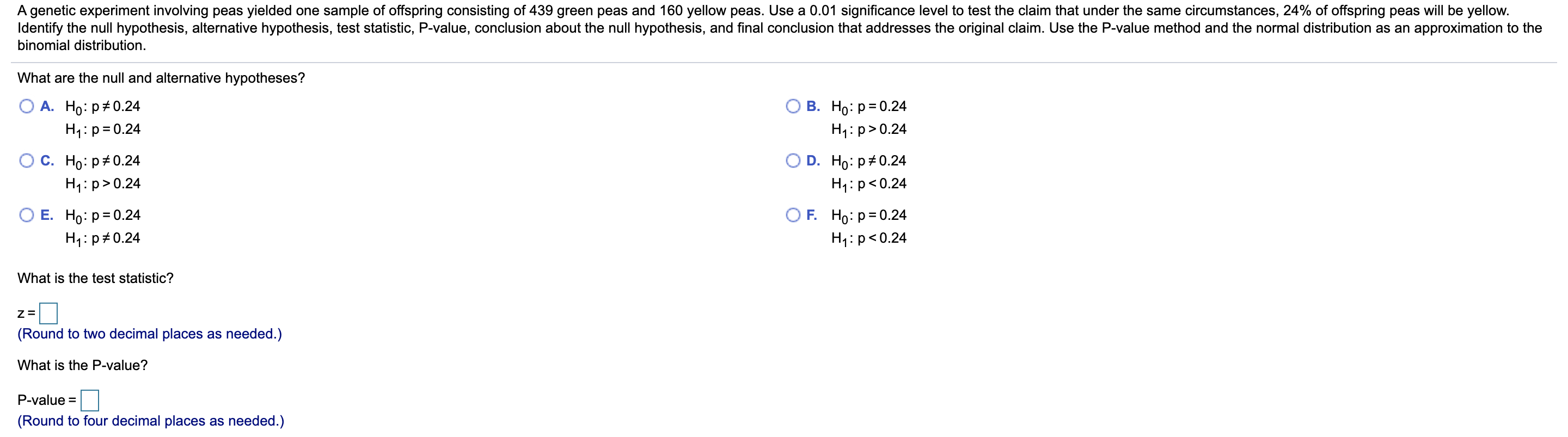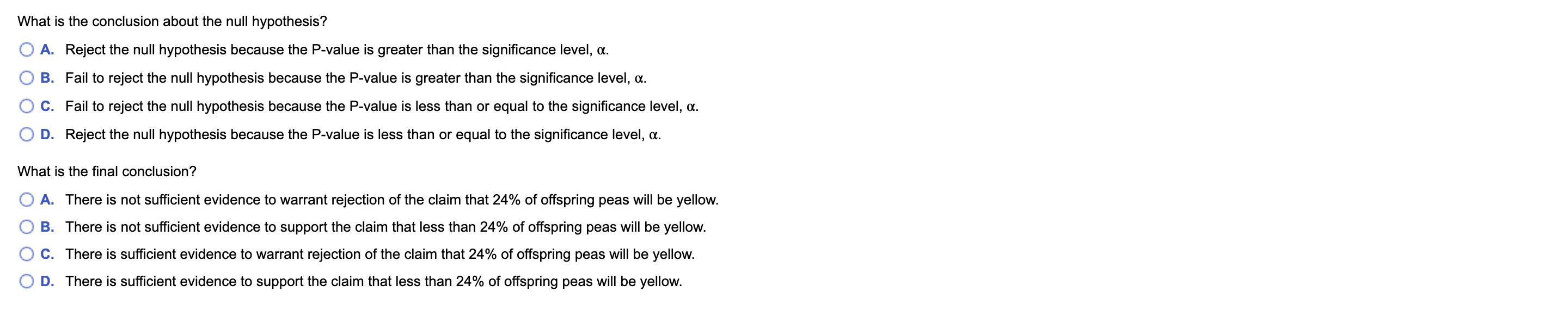# A genetic experiment involving peas yielded one sample of offspring consisting of 439 green peas and 160 yellow peas. Use a 0.01 significance level to test the claim that under the same circumstances, 24% of offspring peas will be yellow.Identify the null hypothesis, alternative hypothesis, test statistic, P-value, conclusion about the null hypothesis, and final conclusion that addresses the original claim. Use the P-value method and the normal distribution as an approximation to thebinomial distribution.What are the null and alternative hypotheses?В. Но: р-0.24H1: p>0.24A. Ho: p+0.24H1:p= 0.24C. Ho: p#0.24O D. Ho: p#0.24H1:p>0.24H1: p

Question
1 viewshelp_outlineImage TranscriptioncloseA genetic experiment involving peas yielded one sample of offspring consisting of 439 green peas and 160 yellow peas. Use a 0.01 significance level to test the claim that under the same circumstances, 24% of offspring peas will be yellow. Identify the null hypothesis, alternative hypothesis, test statistic, P-value, conclusion about the null hypothesis, and final conclusion that addresses the original claim. Use the P-value method and the normal distribution as an approximation to the binomial distribution. What are the null and alternative hypotheses? В. Но: р-0.24 H1: p>0.24 A. Ho: p+0.24 H1:p= 0.24 C. Ho: p#0.24 O D. Ho: p#0.24 H1:p>0.24 H1: p<0.24 O F. Ho: p= 0.24 E. Ho: p= 0.24 H1:p#0.24 H1:p<0.24 What is the test statistic? (Round to two decimal places as needed.) What is the P-value? P-value = (Round to four decimal places as needed.) fullscreenhelp_outlineImage TranscriptioncloseWhat is the conclusion about the null hypothesis? A. Reject the null hypothesis because the P-value is greater than the significance level, a. B. Fail to reject the null hypothesis because the P-value is greater than the significance level, a. C. Fail to reject the null hypothesis because the P-value is less than or equal to the significance level, a. D. Reject the null hypothesis because the P-value is less than or equal to the significance level, a. What is the final conclusion? A. There is not sufficient evidence to warrant rejection of the claim that 24% of offspring peas will be yellow. B. There is not sufficient evidence to support the claim that less than 24% of offspring peas will be yellow. C. There is sufficient evidence to warrant rejection of the claim that 24% of offspring peas will be yellow. D. There is sufficient evidence to support the claim that less than 24% of offspring peas will be yellow. fullscreen
check_circle

Step 1

Given Data

Total peas(n) = green peas + yellow peas = 439 + 160 = 599

Step 2

Formulation of Hypotheses:

The researcher’s objective is to test, whether or not the proportion of yellow offspring peas is equal to 0.24.

The null hypothesis is:

H0­ : p = 0.24

The...help_outlineImage Transcriptionclose0.267 – 0.24 1.547 - 1.55 p(1 – p) 0.24(1 – 0.24) 599 п Calculation of p-value p-value = 2 x P(Z>1.55) =2 X (1- P(Z< 1.55)) = 2 X ( 1-0.9394) = 0.1212 P(Z < 1.55) = 0.9394 ( From Excel =NORM.S.DIST(1.55,TRUE)) || fullscreen

### Want to see the full answer?

See Solution

#### Want to see this answer and more?

Solutions are written by subject experts who are available 24/7. Questions are typically answered within 1 hour.*

See Solution
*Response times may vary by subject and question.
Tagged in
MathStatistics

### Hypothesis Testing